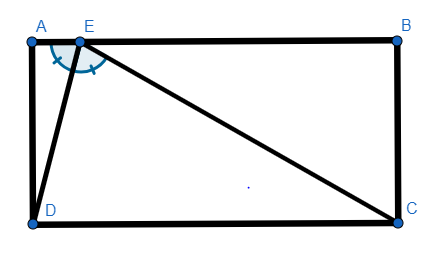# Segments Within Rectangles

Geometry Level 3In rectangle $ABCD$ above, whose length is twice its width, two line segments are drawn from point $E$ to the bottom two vertices $C$ and $D$. If $\overline{DE}$ bisects $\angle{AEC}$, what is the measure, in degrees, of $\angle{AED}$?

×